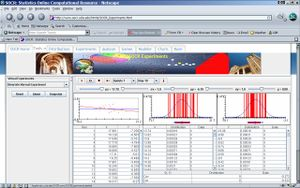# SOCR EduMaterials Activities Bivariate

## SOCR Educational Materials - Activities - SOCR Bivariate Activity

The Bivariate Normal Experiment: The Java applet needed for the following two activities can be found in the SOCR site: http://socr.stat.ucla.edu/htmls/SOCR_experiments.html• Setting: This experiment consists of selecting values for the random variables X and Y which are jointly normally distributed as a bivariate normal f(X,Y) with parameters $$\mu_x=0$$, $$\mu_y=0$$, $$\sigma_x$$=”a value of your choice” , $$\sigma_y$$=”a value of your choice” , and $$\rho$$=”a value of your choice”. The first objective of our activity is to see how the location of the base of the distribution and its spread changes as the parameters change. The second objective is to see how no matter what the values of the parameters are, the marginal distribution of X and the marginal distribution of Y are both normal, with more or less spread depending on the values you assign to the parameters. The points you select on the left hand side diagram, which shows the area above which the normal density lies (or area of integration), can be chosen by setting stop=10,000 update=100 and then clicking on the <RUN> button.

### See what happens when $$\rho$$ and the standard deviation of Y, $$\sigma_y$$, are constant, and the standard deviations of X, $$\sigma_x$$, increases

• Exercise 1: Next, set $$\rho=0.6, \sigma_x=1.3$$ and $$\sigma_y=1.1$$ and select the 10,000 points as indicated above. Write down the mean and standard deviation of the numbers you generated and the correlation. Save the picture.
• Exercise 2: Now, change only the standard deviation of X from 1.3 to 2. Write down the means, standard deviations and correlations of numbers you gnerated. Save the picture.
• Exercise 3: Compare the pictures generated in Exercise 1 and 2 above. What would you say is the effect on the joint distribution $$f(X,Y)$$ of increasing the standard deviation of X, other things held constant. Write your comments here.
• Exercise 4: Compare the marginal densities for X and for Y in Exercise 1 and 2. Which density is more spread out?
• Exercise 5: Compare the regression lines in Exercise 1 and 2. Make your comparison in terms of the slope.

### See what happens when the standard deviations of X and Y are fixed and the correlation increases

• Exercise 6: Fix now the standard deviation of X to 1.3 and the standard deviation of Y to 1.1 and the correlation coefficient to 0.9. Print a screenshot of the pictures, means, standard deviations and correlations in your data. Compare your pictures to those in Exercise 1.
• Exercise 7: According to your results in execrsize 1, what has happened to the joint density function of X and Y as the correlation coefficient has increased? What has happened to the regression line? What has happened to the marginal densities of X and of Y?

### Write here the joint density function of X and Y with parameter values as in Exercise 1

• Exercise 8: Write the formula for the marginal density of X and the marginal density of Y with parameter values as given in Exercise 1. Write the formulas for the conditional densities of X given Y and Y given X, with parameter values as given in Exercise 1. Write then the regression lines that follow from these densities.